# Banach-Jordan pair

(diff) ← Older revision | Latest revision (diff) | Newer revision → (diff)

A real or complex Jordan pair(i.e. a pairof real or complex vector spaces together with trilinear mappings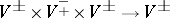,, symmetric in the outer variables, which satisfy: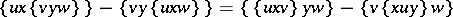for all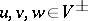,; cf. also Vector space), where the spaces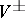are endowed with complete norms (cf. also Norm) making continuous the triple products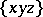,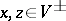,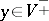. Banach–Jordan triple systems (cf. also Jordan triple system) can be viewed as Banach–Jordan pairswith. For the general theory of Jordan pairs, see [a16]. In the notation of quadratic Jordan theory,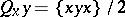. A classic example of a Banach–Jordan pair is that given by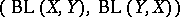, the space of continuous linear operators between two Banach spaces,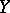, with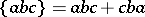(cf. also Banach space; Linear operator). Any Banach–Jordan algebra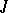gives rise to a Banach–Jordan pair, by taking the usual Jordan triple product. Moreover, Banach–Jordan pairs are interesting in themselves by their connection with bounded symmetric domains [a17]. Jordan–Banach triple systems with additional geometric requirements emerged in the work of W. Kaup. See [a13], [a26] or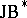-triple for a general report on the theory of-triples (prime-triples have been classified in [a23]); see [a24] for the structure theory of Hilbert triples, Hermitian Hilbert triples and atomic-triples, and see [a8], [a9] for so-called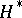-triple theory. A general account of both the geometric and the non-geometric theory of Jordan–Banach triple systems can be found in [a25].

Given a Banach–Jordan pairand an element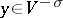,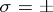, one can define a Banach–Jordan algebra on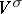, called the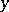-homotope ofand denoted by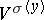, by taking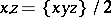as product, and the original norm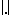of. Of course,can be given an equivalent norm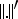so that. The setis a closed ideal of, and so the quotient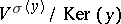is a Banach–Jordan algebra, called the local algebra ofatand usually denoted by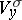. Important notions in the theory of Jordan pairs, such as the Jacobson radical, are defined in terms of homotopes (the adverb "properly" usually refers to properties that hold in all homotopes); on the other hand, local algebras have become a prominent tool in the study of Jordan pairs [a2]. Local algebras play a fundamental role in proving the automatic continuity of derivations on Banach–Jordan pairs [a12], thus extending the extension, by A.R. Villena [a27], of the Johnson–Sinclair theorem to Banach–Jordan algebras.

## Finiteness conditions in Banach–Jordan pairs.

A basic finiteness condition in Jordan pairs is having finite capacity, i.e. the ascending and descending chain conditions on principal inner ideals (cf. also Chain condition). For a non-degenerate Jordan pair(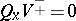implies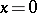,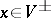), the socle ofcan be characterized as the ideal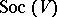of allsuch thathas finite capacity [a22] (cf. also Socle). Notice that for a Jordan pair of type, its socle is the ideal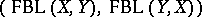of all continuous linear operators having finite rank (cf. also Linear operator). Moreover, it has finite capacity if and only if one of the two vector spaces is finite dimensional.

It is well known that under certain assumptions (von Neumann regularity, finite spectrum, algebraicity or coincidence with the socle) a semi-primitive associative Banach algebra is finite dimensional. This is no longer true for Jordan–Banach algebras, but, under any of the above requirements (in the appropriate Jordan versions), semi-primitive Jordan–Banach algebras have finite capacity [a4]. Using this result and the fact that semi-primitivity is inherited by local algebras, it can be shown (see [a19] and [a14]) that for a semi-primitive complex Banach–Jordan pair, the socle, the set of properly algebraic elements, the largest properly algebraic ideal, the largest properly spectrum-finite ideal, and the largest von Neumann regular ideal all coincide. Related results can be found in [a15], [a20] and [a21]. Since von Neumann regular Banach–Jordan pairs are idempotent-finite, it follows that any von Neumann regular Banach–Jordan pair has finite capacity ([a18] and [a10]). Loos' classification of simple Jordan pairs of finite capacity (any Jordan pair of finite capacity is a direct sum of finitely many simple ones) can be refined in the complex Banach setting: A simple complex Banach–Jordan pair having finite capacity is either finite dimensional, the Jordan pair defined by a continuous symmetric bilinear form on a complex Banach vector space, or a Jordan pair of continuous linear operators between two Banach pairings, one of them being finite dimensional ([a10] and [a11]). Using Zel'manov's structure theorem for strongly prime Jordan systems [a28] (see also [a1]), prime complex Banach–Jordan-triples with non-zero socle and without nilpotent elements were classified in [a6], getting as a consequence, among other results, the Bunce–Chu structure theorem [a7] for compact-triples.

An associative algebra is Noetherian if it satisfies the ascending chain condition on left ideals (cf. also Associative rings and algebras; Noetherian ring). A.M. Sinclair and A.W. Tullo showed [a29] that a complex Noetherian Banach associative algebra is finite dimensional. For a Jordan algebra or pair, the suitable Noetherian condition is the ascending chain condition on inner ideals. M. Benslimane and N. Boudi [a3] proved that a complex Noetherian Banach–Jordan algebra is finite dimensional. This result has been extended, [a5], to Banach–Jordan pairs. Actually, the following has been proved:

i) the Jacobson radical of a Noetherian Banach–Jordan pair is finite dimensional;

ii) non-degenerate Noetherian Banach–Jordan pairs have finite capacity; and

iii) complex Noetherian Banach–Jordan pairs are finite dimensional.

How to Cite This Entry:
Banach-Jordan pair. Encyclopedia of Mathematics. URL: http://encyclopediaofmath.org/index.php?title=Banach-Jordan_pair&oldid=14755
This article was adapted from an original article by Antonio FernÃ¡ndez LÃ³pez (originator), which appeared in Encyclopedia of Mathematics - ISBN 1402006098. See original article